• 模糊c均值聚类python
2020-07-30 16:04:14

模糊C均值聚类的python实现

存在的问题：

用来判断终止条件的变量dist，是前后两次隶属度矩阵的对应元素做差，取绝对值，再取最大值。用鸢尾花数据集的话，dist的值最低只能到小数点后两位，但是网上别的代码能达到小数点后6、7位。

但是标准互信息指数NMI的值相差不大，都在0.74左右。

import numpy as np
from sklearn import metrics
import time

n_row, n_col = data.shape
n_col = n_col - 1
y = np.array(data[:, -1], dtype=np.int)
X = data[:, 0:n_col]

return X, y

def random_membership_matrix(n_sample, k):
# 初始化一个隶属度矩阵
membership_matrix = np.zeros((n_sample, k), dtype=np.float)
for i in range(n_sample):
temp = np.random.random(k)  # 生成k个随机浮点数
temp = temp / sum(temp)
membership_matrix[i] = temp
return membership_matrix

def iterate(X, u, k, m):
# 停止条件来自：https://blog.csdn.net/lyxleft/article/details/88964494
# 前后两次隶属度矩阵对应元素做差，差的最大值不超过某一阈值
n_sample, dim = X.shape
# 先把前一次的隶属度矩阵存起来
old_u = np.array(u)
ter = 0.0000001  # 阈值
iteration = 0
dist = float('inf')
max_iter = 20  # 最大迭代次数，这个次数之内达不到阈值，就得重新随机隶属度矩阵了
center = np.zeros((k, dim), dtype=np.float)  # 类中心，每行存一个类中心

# 满足最小阈值，或者达到最大迭代次数时停止
while dist > ter and iteration < max_iter:

iteration = iteration + 1

# 根据隶属度矩阵求中心
for i in range(k):
up = np.zeros((dim,), dtype=np.float)
down = 0.0
for j in range(n_sample):
up += np.power(u[j, i], m) * X[j]
down += np.power(u[j, i], m)
center[i] = up / down

# 根据中心求隶属度矩阵
for p in range(n_sample):
for q in range(k):
down = 0.0
up = np.power(np.linalg.norm(X[p] - center[q]), -2/(m-1))
for e in range(k):
down += np.power(np.linalg.norm(X[p] - center[e]), -2/(m-1))
u[p, q] = up / down

# 求终止条件
dist = (abs(u - old_u)).max()

return center, u

def cluster(membership_matrix):
# 根据隶属度求聚类结果，把样本点归到隶属度最大的类
n_sample = membership_matrix.shape
y_pred = np.zeros((n_sample,), dtype=np.int)
for i in range(n_sample):
y_pred[i] = np.argmax(membership_matrix[i])
return y_pred

if __name__ == '__main__':
k = 3
m = 2
fileName = 'iris'
X, y = read_txt('./dataset/' + fileName + '.txt')
n_sample, dim = X.shape
# 多跑几次取最好结果
best_y_pred = np.zeros((n_sample,), dtype=np.int)
best_nmi = 0.0
center = np.zeros((k, dim), dtype=np.float)
for d in range(10):
print('**********第%d次随机隶属度矩阵**********' % d)
membership_matrix = random_membership_matrix(n_sample, k)
center, membership_matrix = iterate(X, membership_matrix, k, m)
y_pred = cluster(membership_matrix)
nmi = metrics.normalized_mutual_info_score(y, y_pred)
if nmi > best_nmi:
best_nmi = nmi
best_y_pred = y_pred
print('best_nmi: %f' % best_nmi)
print(center)numpy
更多相关内容
• 模糊C均值聚类Python算法，新数据的类别预测
• Fuzzy C Means - Multi clustering and find center of clusters with Python模糊C均值聚类python实现，用于实现图像分割图像分割
• 这是一个基于python图像分割的模糊c均值算法
• 针对传统的模糊C均值聚类算法（FCM）在图像分割中对噪声十分敏感这一局限性，提出一种自适应的FCM图像分割方法。该方法充分考虑图像像素的灰度信息和空间信息，根据像素的空间位置自适应地计算一个合适的相似度距离...
• 模糊聚类该软件包实现了模糊c均值（FCM）分类算法，以及用于可视化分类结果的一组图形工具。 FCM执行软分类。 不是将样本分配给单个类别，而是为每个样本赋予每个类别一个成员资格评分（类似于归属概率）。 该算法...
•   模糊c均值聚类(Fuzzy C-Means)是引入了模糊理论的一种聚类算法，通过隶属度来表示样本属于某一类的概率，原因在于在很多情况下多个类别之间的界限并不是绝对的明确。显然，相比于k-means的硬聚类，模糊c均值聚类...

#### 原理简介

模糊c均值聚类(Fuzzy C-Means)是引入了模糊理论的一种聚类算法，通过隶属度来表示样本属于某一类的概率，原因在于在很多情况下多个类别之间的界限并不是绝对的明确。显然，相比于k-means的硬聚类，模糊c均值聚类得到的聚类结果更灵活。

模糊c均值聚类通过最小化一下目标函数来得到聚类中心：

J m = ∑ i = 1 N ∑ j = 1 C u i j m ∥ x i − c j ∥ 2 , 1 ≤ m < ∞ (1) J_{m}=\sum_{i=1}^{N} \sum_{j=1}^{C} u_{i j}^{m}\left\|x_{i}-c_{j}\right\|^{2} \quad, \quad 1 \leq m<\infty \tag{1}

其中， m > 1 m>1 为模糊系数(fuzzy coefficient)， N N 为样本数， C C 为聚类中心数， c j c_j 表示第 j j 个聚类中心，和样本特征维数相同， x i x_i 表示第 i i 个样本， u i j u_{ij} 表示样本 x i x_i 对聚类中心 c j c_j 的隶属度(通俗的说就是 x i x_i 属于 c j c_j 的概率)，显然满足

∑ j = 1 C u i j = 1 (2) \sum_{j=1}^{C} u_{i j}=1 \tag{2}

∣ ∣ ∗ ∣ ∣ ||*|| 可以是任意度量数据相似性(距离)的范数，最常见的就是欧几里得范数（又称欧氏范数，L2范数，欧氏距离）：

d = ∥ x ∥ 2 = ∑ i x i 2 (3) d=\|x\|_2=\sqrt{\sum_i {x_i^2}} \tag{3}

模糊c均值聚类通过更新 u i j u_{ij} c j c_j 来迭代地优化目标函数Eq. (1)：

u i j = 1 ∑ k = 1 C ( ∥ x i − c j ∥ ∥ x i − c k ∥ ) 2 m − 1 (4) u_{i j}=\frac{1}{\sum_{k=1}^{C}\left(\frac{\left\|x_{i}-c_{j}\right\|}{\left\|x_{i}-c_{k}\right\|}\right)^{\frac{2}{m-1}}} \tag{4}

c j = ∑ i = 1 N u i j m ⋅ x i ∑ i = 1 N u i j m (5) c_{j}=\frac{\sum_{i=1}^{N} u_{i j}^{m} \cdot x_{i}}{\sum_{i=1}^{N} u_{i j}^{m}} \tag{5}

迭代的终止条件为 max ⁡ i j { ∣ u i j ( t + 1 ) − u i j ( t ) ∣ } < ε \max _{ij}\left\{\left|u_{ij}^{(t+1)}-u_{ij}^{(t)}\right|\right\}<\varepsilon ，其中 t t 是迭代步数， ε \varepsilon 是一个很小的常数表示误差阈值。也就是说迭代地更新 u i j u_{ij} c j c_j 直到前后两次隶属度最大变化值不超过误差阈值。这个过程最终收敛于 J m J_m 的局部极小值点或鞍点

#### 算法步骤

可以将模糊c均值聚类的过程归纳为以下几步：

1. 初始化隶属度矩阵 U ( 0 ) U^{(0)} ，若有 N N 个样本，指定类别数为 C C ，则隶属度矩阵应当是 N ∗ C N*C 的矩阵；
2. 根据式(5)更新聚类中心 c j , j = 1 , . . . , C c_j, j=1,...,C
3. 根据式(4)更新 U ( t ) , U ( t + 1 ) U^{(t)}, U^{(t+1)}
4. 若满足终止条件 max ⁡ i j { ∣ u i j ( t + 1 ) − u i j ( t ) ∣ } < ε \max _{ij}\left\{\left|u_{ij}^{(t+1)}-u_{ij}^{(t)}\right|\right\}<\varepsilon 则停止迭代，否则返回步骤2。

#### 程序实现

下面代码以Iris数据集聚类为例实现了fuzzy c-means。

#!/usr/bin/python3
# -*- coding: utf-8 -*-

'''
@Date    : 2019/9/11
@Author  : Rezero
'''

import numpy as np
import pandas as pd

data = data.sample(frac=1.0)   # 打乱数据顺序
dataX = data.iloc[:, :-1].values # 特征
labels = data.iloc[:, -1].values # 标签
# 将标签类别用 0, 1, 2表示
labels[np.where(labels == "Iris-setosa")] = 0
labels[np.where(labels == "Iris-versicolor")] = 1
labels[np.where(labels == "Iris-virginica")] = 2

return dataX, labels

def initialize_U(samples, classes):
U = np.random.rand(samples, classes)  # 先生成随机矩阵
sumU = 1 / np.sum(U, axis=1)   # 求每行的和
U = np.multiply(U.T, sumU)   # 使隶属度矩阵每一行和为1

return U.T

# 计算样本和簇中心的距离，这里使用欧氏距离
def distance(X, centroid):
return np.sqrt(np.sum((X-centroid)**2, axis=1))

def computeU(X, centroids, m=2):
sampleNumber = X.shape  # 样本数
classes = len(centroids)
U = np.zeros((sampleNumber, classes))
# 更新隶属度矩阵
for i in range(classes):
for k in range(classes):
U[:, i] += (distance(X, centroids[i]) / distance(X, centroids[k])) ** (2 / (m - 1))
U = 1 / U

return U

def ajustCentroid(centroids, U, labels):
newCentroids = [[], [], []]
curr = np.argmax(U, axis=1)  # 当前中心顺序得到的标签
for i in range(len(centroids)):
index = np.where(curr == i)   # 建立中心和类别的映射
trueLabel = list(labels[index])  # 获取labels[index]出现次数最多的元素，就是真实类别
trueLabel = max(set(trueLabel), key=trueLabel.count)
newCentroids[trueLabel] = centroids[i]
return newCentroids

def cluster(data, labels, m, classes, EPS):
"""
:param data: 数据集
:param m: 模糊系数(fuzziness coefficient)
:param classes: 类别数
:return: 聚类中心
"""
sampleNumber = data.shape  # 样本数
cNumber = data.shape       # 特征数
U = initialize_U(sampleNumber, classes)   # 初始化隶属度矩阵
U_old = np.zeros((sampleNumber, classes))

while True:
centroids = []
# 更新簇中心
for i in range(classes):
centroid = np.dot(U[:, i]**m, data) / (np.sum(U[:, i]**m))
centroids.append(centroid)

U_old = U.copy()
U = computeU(data, centroids, m)  # 计算新的隶属度矩阵

if np.max(np.abs(U - U_old)) < EPS:
# 这里的类别和数据标签并不是一一对应的, 调整使得第i个中心表示第i类
centroids = ajustCentroid(centroids, U, labels)
return centroids, U

# 预测所属的类别
def predict(X, centroids):
labels = np.zeros(X.shape)
U = computeU(X, centroids)  # 计算隶属度矩阵
labels = np.argmax(U, axis=1)  # 找到隶属度矩阵中每行的最大值，即该样本最大可能所属类别

return labels

def main():
datapath = "iris.data"
dataX, labels = loadData(datapath)  # 读取数据

# 划分训练集和测试集
ratio = 0.6  # 训练集的比例
trainLength = int(dataX.shape * ratio)  # 训练集长度
trainX = dataX[:trainLength, :]
trainLabels = labels[:trainLength]
testX = dataX[trainLength:, :]
testLabels = labels[trainLength:]

EPS = 1e-6   # 停止误差条件
m = 2        # 模糊因子
classes = 3  # 类别数
# 得到各类别的中心
centroids, U = cluster(trainX, trainLabels, m, classes, EPS)

trainLabels_prediction = predict(trainX, centroids)
testLabels_prediction = predict(testX, centroids)

train_error = 1 - np.sum(np.abs(trainLabels_prediction - trainLabels)) / trainLength
test_error = 1 - np.sum(np.abs(testLabels_prediction - testLabels)) / (dataX.shape - trainLength)
print("Clustering on traintset is %.2f%%" % (train_error*100))
print("Clustering on testset is %.2f%%" % (test_error*100))

if __name__ == "__main__":
main()


#### 参考资料

展开全文• 模糊”：一个元素可以不同程度的属于某几个子集，也就是说元素对于集合的隶属度可以在[0,1]上取连续值。翻译一下： S1：初始化参数：加权指数m，簇心数目C，以及迭代停止阈值ε。S2：随机初始化隶属度矩阵U，注意...

# 一、模糊

“模糊”：一个元素可以不同程度的属于某几个子集，也就是说元素对于集合的隶属度可以在[0,1]上取连续值。

# 二、步骤

## 2.1步骤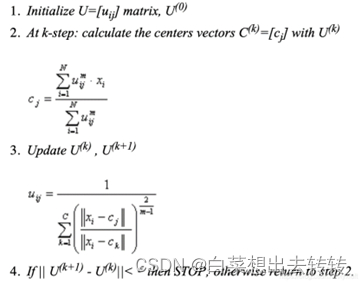翻译一下：

S1：初始化参数：加权指数m，簇心数目C，以及迭代停止阈值ε

S2：随机初始化隶属度矩阵U，注意满足式（2-2）。

S3：式（2-3）更新簇心c。

S4：式（2-4）更新隶属度矩阵U

S5：如果隶属度矩阵U满足式（2-5）则返回U并结束算法，否则转到S2

各种式如下：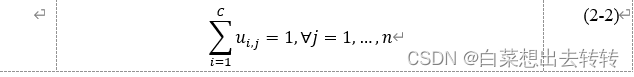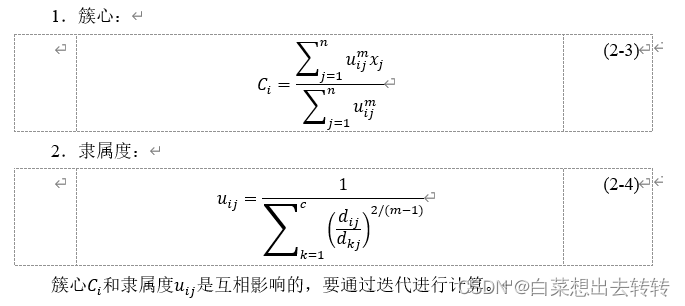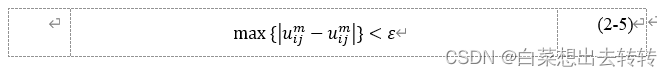## 2.2流程图

流程图比步骤多的一项是计算目标函数J，这一步可有可无，为了观察目标函数的变化趋势，我们在流程图和代码里都加上了计算目标函数这一步。

# 三、代码

import numpy as np
import matplotlib.pyplot as plt
import time
star = time.time()  # 计时
row = img.shape
col = img.shape
plt.figure(1)
plt.subplot(221)
plt.imshow(img)

def fcm(data, threshold, k, m):
# 0.初始化
data = data.reshape(-1, 3)
cluster_center = np.zeros([k, 3])  # 簇心
distance = np.zeros([k, row*col])  # 欧氏距离
times = 0  # 迭代次数
goal_j = np.array([])  # 迭代终止条件：目标函数
goal_u = np.array([])  # 迭代终止条件：隶属度矩阵元素最大变化量
# 1.初始化U
u = np.random.dirichlet(np.ones(k), row*col).T  # 形状（k, col*rol），任意一列元素和=1
#  for s in range(50):
while 1:
times += 1
print('循环：', times)
# 2.簇心update
for i in range(k):
cluster_center[i] = np.sum((np.tile(u[i] ** m, (3, 1))).T * data, axis=0) / np.sum(u[i] ** m)
# 3.U update
# 3.1欧拉距离
for i in range(k):
distance[i] = np.sqrt(np.sum((data - np.tile(cluster_center[i], (row * col, 1))) ** 2, axis=1))
# 3.2目标函数
goal_j = np.append(goal_j, np.sum((u**m)*distance**2))
# 3.3 更新隶属度矩阵
oldu = u.copy()  # 记录上一次隶属度矩阵
u = np.zeros([k, row * col])
for i in range(k):
for j in range(k):
u[i] += (distance[i] / distance[j]) ** (2 / (m - 1))
u[i] = 1/u[i]
goal_u = np.append(goal_u, np.max(u - oldu))  # 隶属度元素最大变化量
print('隶属度元素最大变化量', np.max(u - oldu), '目标函数', np.sum((u**m)*distance**2))
# 4.判断：隶属度矩阵元素最大变化量是否小于阈值
if np.max(u - oldu) <= threshold:
break
return u, goal_j, goal_u

if __name__ == '__main__':

img_show, goal1_j, goal2_u = fcm(img, 1e-09, 5, 2)
img_show = np.argmax(img_show, axis=0)
# plt.figure(2)
plt.subplot(223)
plt.plot(goal1_j)
plt.rcParams['font.sans-serif'] = ['SimHei']
plt.rcParams['axes.unicode_minus'] = False
plt.title('目标函数变化曲线')
plt.xlabel('迭代次数')
plt.ylabel('目标函数')
# plt.figure(3)
plt.subplot(224)
plt.plot(goal2_u)
plt.title('隶属度矩阵相邻两次迭代的元素最大变化量变化曲线')
plt.xlabel('迭代次数')
plt.ylabel('隶属度矩阵相邻两次迭代的元素最大变化量')
# plt.figure(1)
plt.subplot(222)
plt.imshow(img_show.reshape([row, col]))
end = time.time()
print('用时：', end - star)
plt.show()


# 四、代码怎么用？

第一步，需要把待分割图片放入项目文件，like this: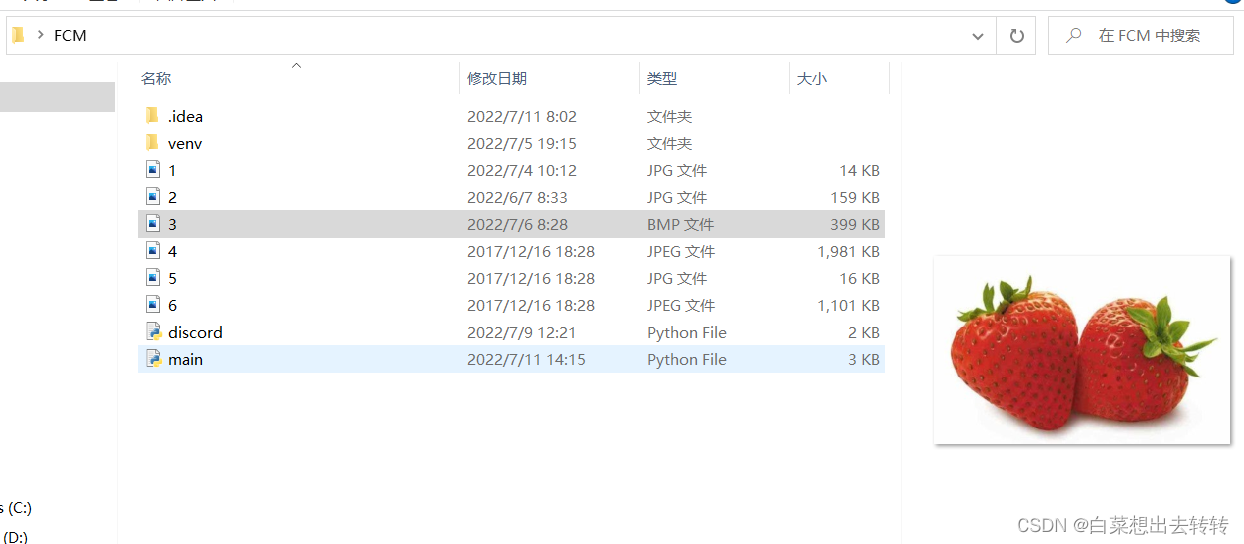# 五、运行结果

1.迭代终止条件的阈值：1e-09；簇总数：5；m=2，迭代了114次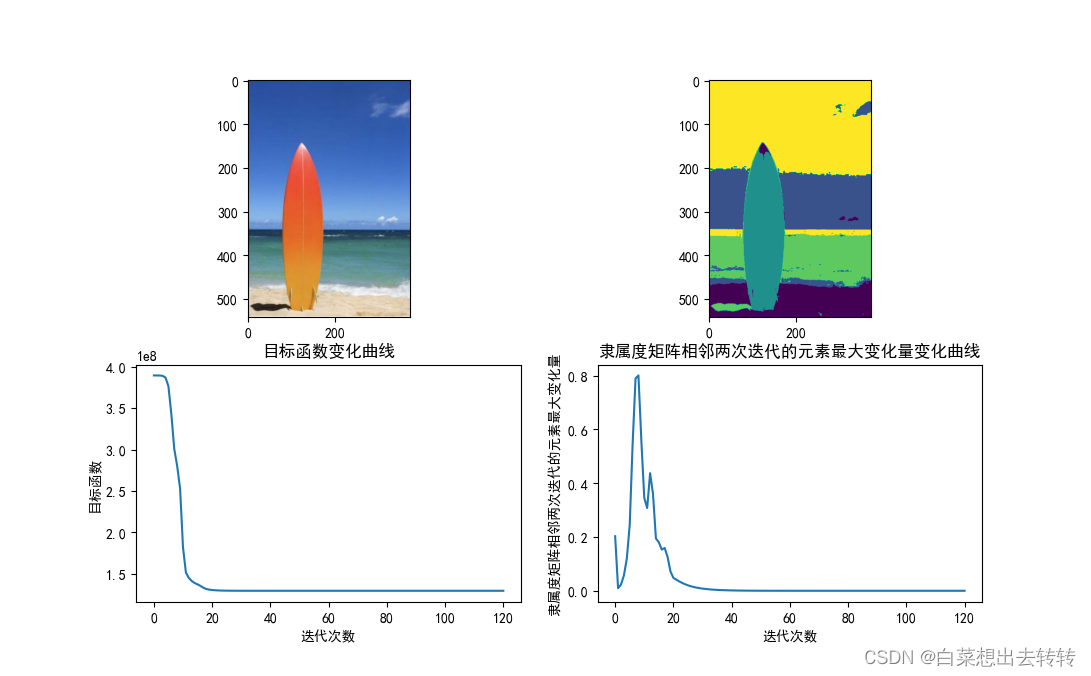2. 迭代终止条件：1e-09；簇总数：2；m=2，迭代了16次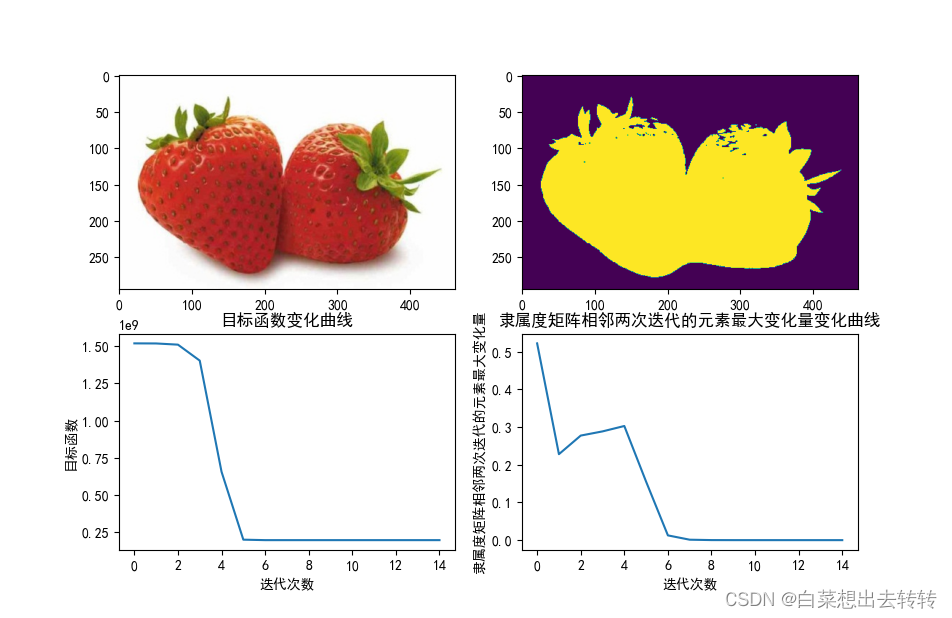3.迭代终止条件：1e-09；簇总数：5；m=2，迭代了170次，用时2306s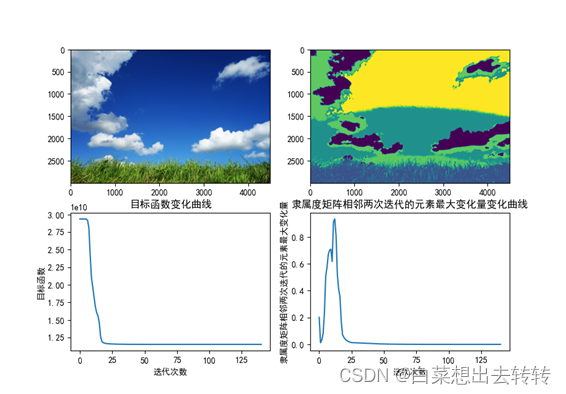# 六、终止条件为目标函数变化值小于阈值：

## 6.1步骤

S1：初始化参数：加权指数m，簇心数目C，以及迭代停止阈值ε

S2：随机初始化隶属度矩阵U，注意满足式（2-2）。

S3：式（2-3）更新簇心c。

S4：式（2-1）计算目标函数，如果变化值小于阈值，则返回U并结束算法，否则转到S2

S5：式（2-4）更新隶属度矩阵U

## 6.2 流程图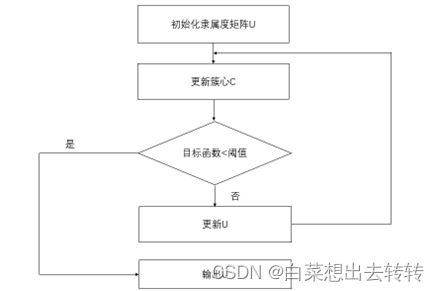# 七、代码详解

## 7.1产生和为1的随机数

### 7.1.1德雷克分布

产生(m ,n)的二维数组，每行元素和=1

import numpy as np
x = np.random.dirichlet(np.ones(n), size=m)


### 7.1.2返回值

形状：(1, 20),二维数组。

转换为向量：x=x

类型：ndarray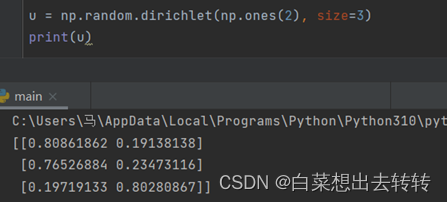## 7.2三维数组

### 7.2.1三维和二维

形状(k, m, n)的三维数组，相对于k个形状(m, n)的二维数组

### 7.2.2索引二维数组

三维数组arr，形状（k,m,n）。arr第1个二维数组为arr

## 7.3未知形状数组加入新值

### 7.3.1应用场景

在某一循环内，在循环的总次数不清楚的情况下，需要记录每次循环的结果。即需要给一个不清楚长度数组加入元素。

比如，在代码中，每次循环都记录目标函数的值，但是循环的总次数每次都不确定。

### 7.3.2实现

先定义x是一个空数组，再通过x.append()加入新值。

x = np.array([])
for i in range(n):
…
x.append(data)


还有一些关于语法的解释，可以看下面这篇，虽然是kmeans算法但是编程上原理差不多。

展开全文数据挖掘
• 目录一、理论介绍二、算法实现思路三、FCM算法核心代码的python实现四、使用示例1.对数据进行聚类2.图片分割五、实现时出现的错误总结六、源码链接 一、理论介绍 二、算法实现思路 下面给出该算法的基本思路： ...

这篇文章是楼主老早之前做课后作业写的，如果有问题的话建议米娜桑自行解决哦~

## 一、理论介绍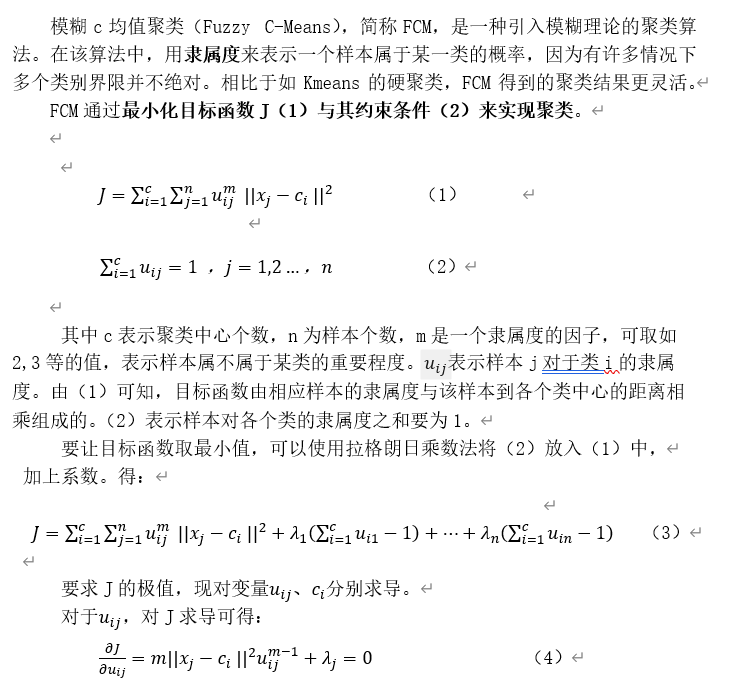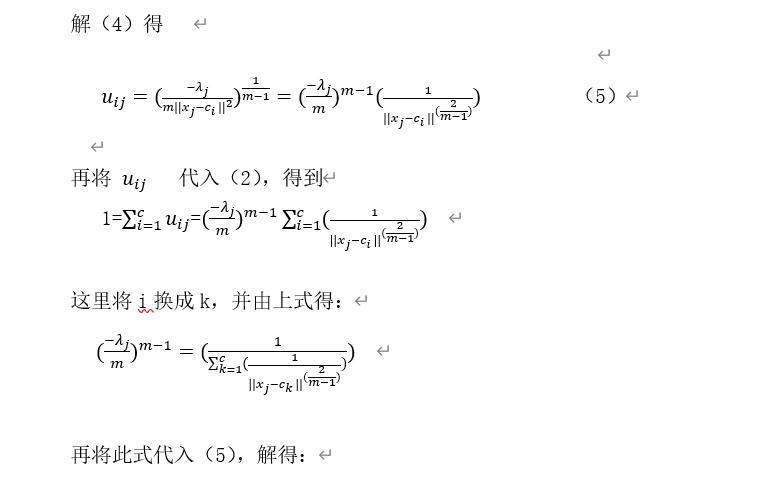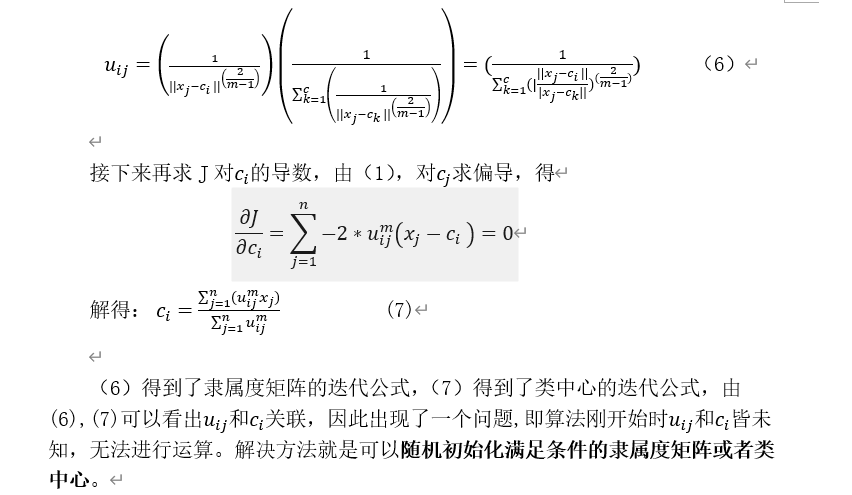## 二、算法实现思路

下面给出该算法的基本思路：
1.可以先初始化一个隶属度矩阵U（每个样本对各个类的隶属度和为1）。
2.根据U开始对类中心矩阵C进行计算。
3.再根据C计算U。
4.循环2,3步骤直到满足循环条件，结束。

## 三、FCM算法核心代码的python实现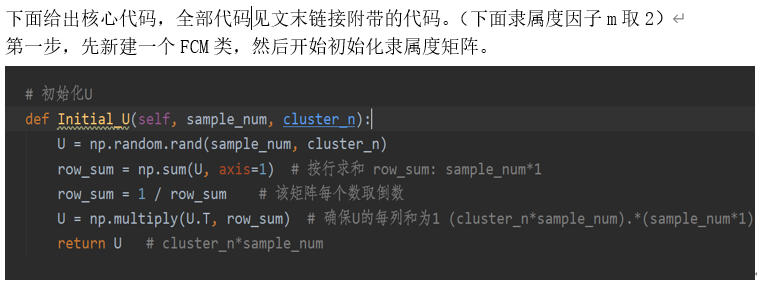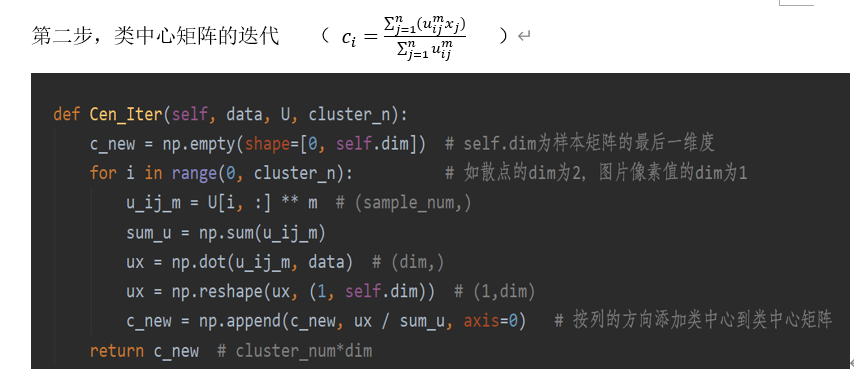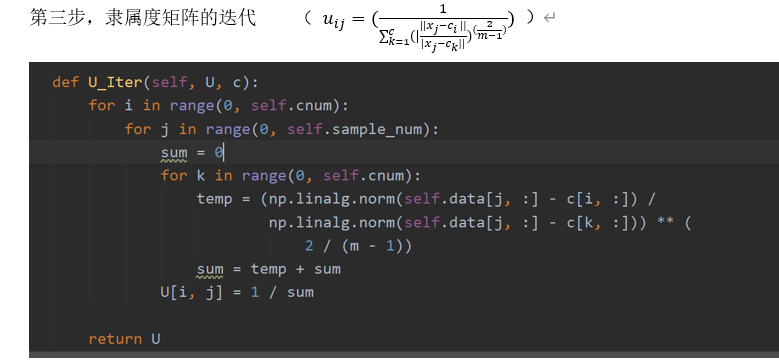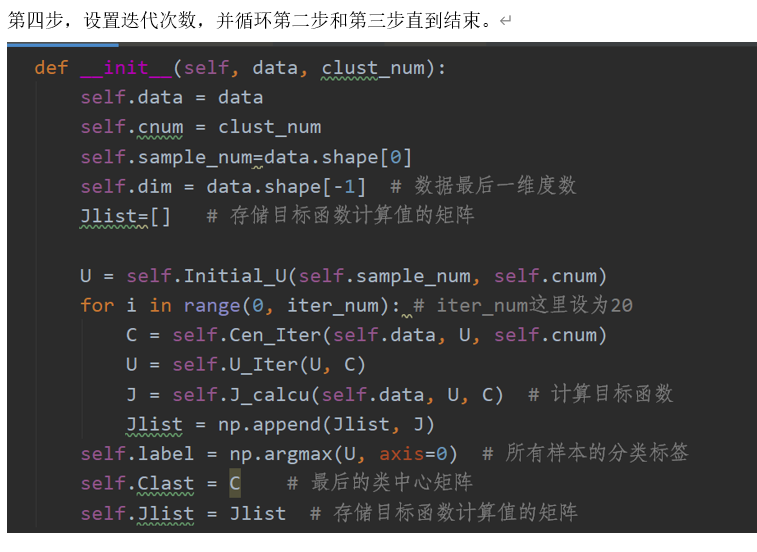## 四、使用示例

### 1.对数据进行聚类

下面我用np的random生成一些数据来对FCM进行展示
示例1：示例2：示例3：### 2.图片分割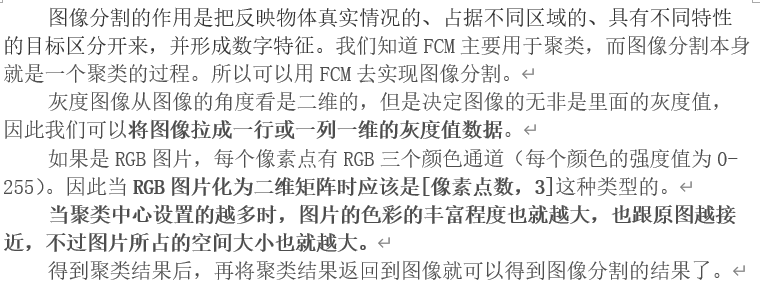下面展示图片分割的结果~

灰度图：（下面展示聚类中心为2和15两种）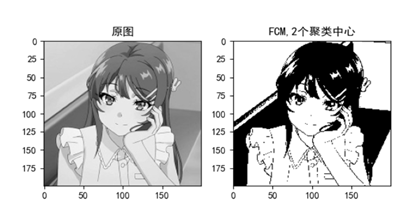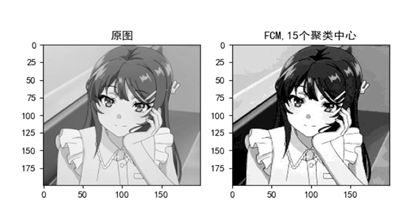Ps:聚类中心不要设太大，图片不要太大！！！

彩图：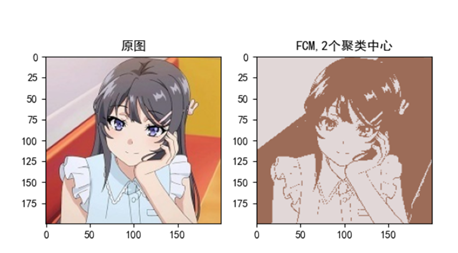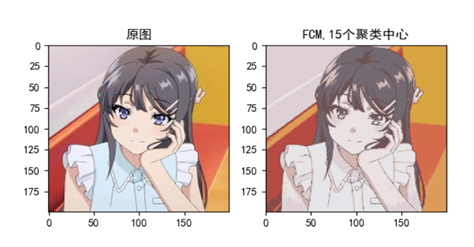可以看到，分割后的图片还是能有清晰的轮廓，只是灰度或色彩的种类数减少了。

## 五、实现时出现的错误总结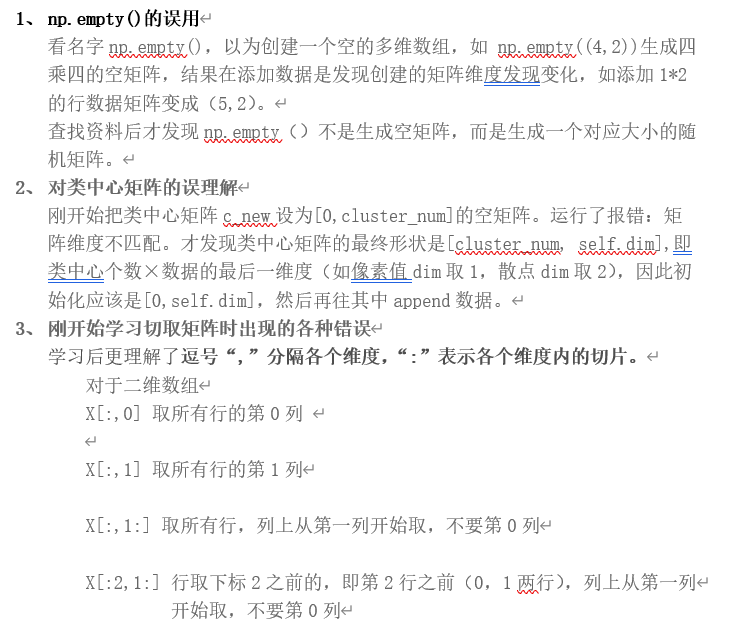## 六、源码链接

点击此处，混合食用最佳

展开全文• 关于算法的原理，大家可以看这篇博客：聚类分析之模糊C均值算法核心思想，注意博客中有关隶属度计算的公式有点小问题，这里更正如下： Wij=(1/dist⁡(xi,cj)2)1p−1/∑q=1k(1/dist⁡(xi,cq)2)1p−1W _ { i j } = ( 1...算法 机器学习 大数据
• 准备说明：Python代码运行，需要有数据集，文章最后有csv格式的数据集，请自行下载。 理论知识讲解： 模糊理论 模糊控制是自动化控制领域的一项经典方法。其原理则是模糊数学、模糊逻辑。1965，L. A. Zadeh...机器学习
•     模糊c均值聚类融合了模糊理论的精髓。相较于k-means的硬聚类，模糊c提供了更加灵活的聚类结果。因为大部分情况下，数据集中的对象不能划分成为明显分离的簇，指派一个对象到一个特定的簇有些生硬，也可能会...算法
• ## 模糊c均值聚类算法原理详细讲解

万次阅读 多人点赞 2020-11-29 15:27:53
模糊c均值聚类算法详细讲解python（一）聚类和模糊简述(二）模糊c均值聚类原理（1）目标函数（三）模糊c均值聚类算法步骤 （一）聚类和模糊简述 聚类分析是多元统计分析的一种，也是无监督模式识别的一个重要分支，...算法
• 目录模糊理论Fuzzy C-Means算法原理算法步骤python实现参考资料本文采用数据集为iris,将iris.txt放在程序的同一文件夹下。请先自行下载好。模糊理论模糊控制是自动化控制领域的一项经典方法。其原理则是模糊数学、...
• python3模糊C均值聚类算法，数据集为iris，放在和代码同一目录即可。算法原理及步骤参考：https://wenku.baidu.com/view/ee968c00eff9aef8941e06a2.html机器学习
• python语言FCM（模糊C均值聚类）第三方包介绍数据挖掘
• 聚类算法及python实现——模糊C均值(FCM) 模糊C和K均值的区别 K均值：硬聚类，隶属度只有0和1，基于“类内误差平方和最小化”原则 模糊C：模糊聚类，隶属度取值为[0，1]，基于“类内加权误差平方和最小化”原则 步骤...
• ：图像分割是基于内容的服装图像检索系统的重要研究内容之一，传统的模糊Ｃ均值聚类算法在用于图像分割时，存在对样本分布和输入参数有限制、收敛于局部最优解、对噪声敏感等缺点．本文加入高斯核函数优化空间样本...
• .模糊理论的介绍 在日常生活中，有许多事物或多或少都具有模糊性，模糊虽难以捉摸，但却非常...故模糊理论将模糊概念以模糊集合的定义，将事件属于某集合程度的隶属函数加以模糊量化，得到隶属度，来处理问题。 ...算法 机器学习 深度学习
• 模糊C均值聚类算法是一种常用的无监督分类方法，广泛的应用于数据挖掘领域中，主要融合了K均值聚类算法和模糊理论，算法步骤如下所示： Python实现 import torch import random import numpy as np from sklearn ...
• 计算Lyapunov指数的模糊C均值聚类小数据量法.pdf
• 模式识别学习，课程实例分享。...其中，C均值算法聚类准则是以最小欧式距离方法，将每个点分配到最近的聚类中来实现的。 本节主要分成两个测试小节来描述C均值算法的特性，并用三维图像模型对聚类效果进行直观展示...代码实例 理论分析 模式识别
• 模糊理论模糊控制是自动化控制领域的一项经典方法。其原理则是模糊数学、模糊逻辑。1965，L. A. Zadeh发表模糊集合“Fuzzy Sets”的论文， 首次引入隶属度函数的概念，打破了经典数学“非0即 1”的局限性，用[0,1]...
• ## FCM模糊聚类算法python实现

千次阅读 热门讨论 2020-05-26 20:08:20
FCM原理 FCM隶属度矩阵以及聚类中心的更新关系可以参考这个文章，推倒过程已经很详细了，本博客在理论的基础上对公式进行解读和变换使之对应到相应的矩阵操作，并最终完成模糊聚类算法的编写。...公式解读 ...算法 机器学习
• FCM(fuzzy c-means)模糊c均值聚类融合了模糊理论的精髓。相较于k-means的硬聚类，模糊c提供了更加灵活的聚类结果。因为大部分情况下，数据集中的对象不能划分成为明显分离的簇，指派一个对象到一个特定的簇有些生硬...
• den = sum([math.pow(float(distances[j]/distances[c]), 2) for c in range(k)]) membership_mat[i][j] = float(1/den) return membership_mat,data #得到聚类结果 def getClusters(membership_mat): cluster......